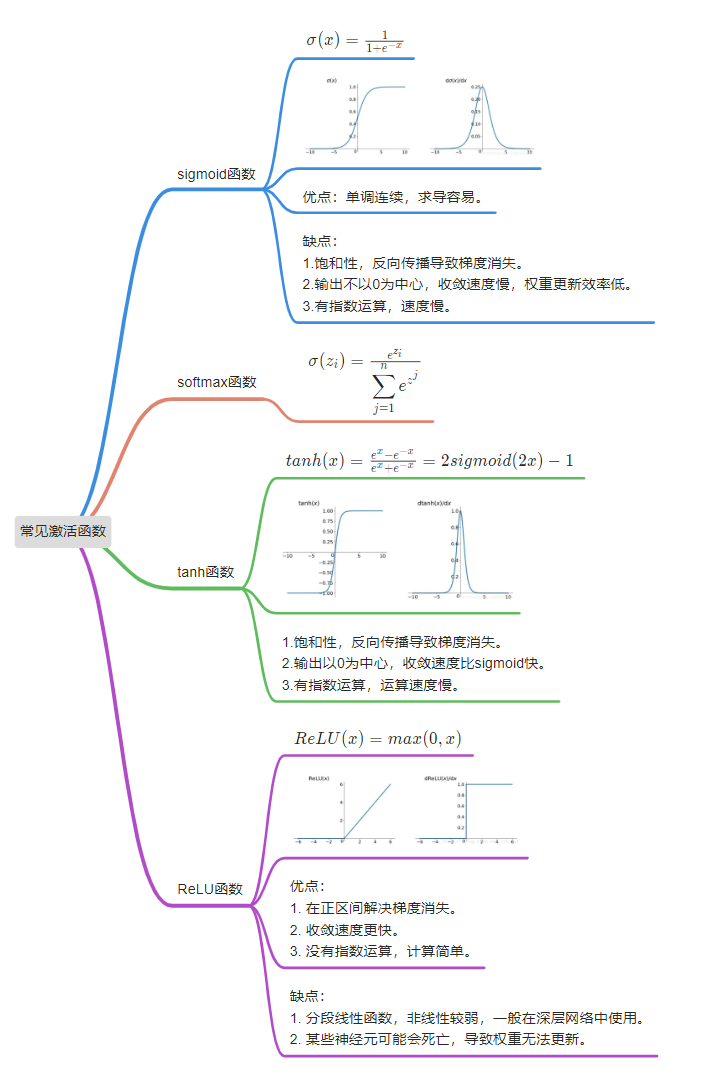• 常见激活函数 激活函数以0为中心的收敛解释 反向传播过程中，用链式法则求导，若连乘数字都小于1，则梯度越乘越小，导致梯度消失。若连乘数字大于1，则梯度越乘越大，导致梯度爆炸。
常见激活函数
激活函数以0为中心的收敛解释
反向传播过程中，用链式法则求导，若连乘数字都小于1，则梯度越乘越小，导致梯度消失。若连乘数字大于1，则梯度越乘越大，导致梯度爆炸。展开全文机器学习 神经网络 深度学习
• 常见激活函数激活函数的作用非线性激活函数sigmodtanh 激活函数relu 激活函数 Rectified Linear UnitsLrelu&PreluELUSwishMaxout参考blog 激活函数的作用 激活函数的主要功能是为神经网络非线性能力 ，去掉激活...
常见激活函数激活函数的作用非线性激活函数sigmodtanh 激活函数relu 激活函数 Rectified Linear UnitsLrelu&PreluELUSwishMaxout参考blog
激活函数的作用
激活函数的主要功能是为神经网络非线性能力 ，去掉激活函数层，神经网络仅剩下线性函数，多层线性函数的拟合还是线性的，无法更好的建模生活中的大多数非线性问题。
线性激活函数（不是重点）
非线性激活函数（sigmod,tanh,relu,lrelu,Prelu,swish）
考量：

可微性：因为优化方法是基于梯度的，
单调性：当激活函数是单调的时候，能够保证单层网络是凸函数
输出值的范围：激活函数的输出值的范围可以有限也可以无限。当输出值是有限的时候，基于梯度的优化方法会更加稳定，因为特征的表示受有限权值的影响更加显著；当输出值是无限的时候，模型的训练会更加高效，不过在这种情况下，一般需要更小的learning rate

非线性激活函数
sigmod
公式
$f(x) = \frac{1}{1+e^{-x}} \\ f^{'}(x) = f(x)*(1-f(x))$
性质不错，可做归一化和表示为概率
左端趋近于0，右端趋近与1，两边饱和性 ，两边导数趋近于0
当输入落入饱和区，梯度比较小，参数更新难度变大，此现象为梯度消失 。
激活函数的偏移现象。sigmoid函数的输出值均大于0，使得输出不是0的均值，这会导致后一层的神经元将得到上一层非0均值的信号作为输入。
tanh 激活函数
$tanh = \frac{1-e^{-2x}}{1+e^{-2x}}$
解决了Sigmoid函数的不是zero-centered输出问题,
使得它的收敛速度要比sigmoid快，减少了迭代更新的次数。然而，梯度消失（gradient vanishing）的问题和幂运算的问题仍然存在
relu 激活函数 Rectified Linear Units
$f(x) = max(0,x)$
优点：
1） 解决了gradient vanishing问题 (在正区间)
2）计算速度非常快，只需要判断输入是否大于0
3）收敛速度远快于sigmoid和tanh
缺点：
1）ReLU的输出不是zero-centered
2）某些神经元可能永远不会被激活(Dead ReLU Problem)，导致相应的参数永远不能被更新
初始化方法
学习率太高
Lrelu&Prelu
$f(x) = x ,x>0 \\ f(x) = \alpha*x,x<=0$
针对 x<0 的硬饱和问题，我们对 [公式] 作出改进，提出Leaky-ReLU，即在 x<0 部分添加一个参数 \alpha。
P-ReLU则认为 [公式] 也应当作为一个参数来学习，一般建议 \alpha 初始化为0.25
ELU
$f(x) = x ,x>0 \\ f(x) = \alpha*(e^x-1)$
LU是结合了sigmoid的左侧软饱和性和ReLU的右侧无饱和性而提出的一种新的激活函数。从上面图中不难看到这一特点。右侧线性部分使得ELU可以缓解梯度消失问题，而左侧软饱和性能让ELU对输入变化或噪声更鲁棒。
Swish
$swish(x) = x*sigmod$
Swish函数跟ReLu差不多，唯一区别较大的是接近于0的负半轴区域, Google大脑做了很多实验，结果都表明Swish优于ReLu
Maxout
$Maxout: f(x) = max(w_1^tx+b_1，...,w_n^tx+b_n)$
maxout网络能够近似任意连续函数，且当 [公式] 为0时，退化为ReLU。Maxout能够缓解梯度消失，同时又规避了ReLU神经元死亡的缺点，但增加了参数和计算量
。。。留待更新
参考blog
https://zhuanlan.zhihu.com/p/70810466
https://baijiahao.baidu.com/s?id=1653421414340022957&wfr=spider&for=pc


展开全文深度学习
• 深度学习常见激活函数的特点
sigmoid：输入值很大时对应的函数值接近1或0，处于函数的饱和区，导致梯度几乎为0，造成梯度消失问题
Relu：解决梯度消失问题，但是会出现dying relu现象，即训练过程中，有些神经元实际上已经"死亡“而不再输出任何数值
Leaky Relu：f = max(αx, x)，解决dying relu问题，α的取值较大时比较小时的效果更好。它有一个衍生函数，parametric Leaky Relu，在该函数中α是需要去学习的
ELU：避免dying神经元，并且处处连续，从而加速SGD，但是计算比较复杂
激活函数的选择顺序：ELU>Leaky Relu及其变体>Relu>tanh>sigmoid

转自微信公众号，忘记哪篇文章了，如有原作看到，欢迎联系。


展开全文深度学习
• 常见激活函数阶跃型函数sgnsgnsgn函数SigmoidSigmoidSigmoid型函数LogisticLogisticLogistic函数TanhTanhTanh函数Hard−LogisticHard-LogisticHard−Logistic函数和Hard−TanhHard-TanhHard−Tanh函数Hard−...
常见激活函数阶跃型函数$sgn$函数$Sigmoid$型函数$Logistic$函数$Tanh$函数$Hard-Logistic$函数和$Hard-Tanh$函数$Hard-Logistic$函数$Hard-Tanh$函数$ReLU$函数带泄露的$ReLU$带参数的$ReLU$$ELU$函数$Softplus$函数$Swish$函数高斯误差线性单元$Maxout$单元
阶跃型函数
$sgn$函数
$sgn$函数定义为：
$sgn(x) = \begin{cases} 1, & x \geq 0; \\ 0, & x< 0. \end{cases}$
$sgn(x)$通过将输入转换为输出值$0$和$1$，来表示神经元的抑制($0$)和兴奋($1$)。然而，由于$sgn(x)$具有不连续、不光滑等不太好的性质，因此实际常用$Sigmoid$型函数做为激活函数。
$Sigmoid$型函数
$Sigmoid$型函数是指一类$S$型曲线函数，为两端饱和函数。常用的$Sigmoid$型函数有$Logistic$函数和$Tanh$函数。

饱和
对于函数$f(x)$，若$x \rightarrow -\infty$时，其导数$f^\prime(x) \rightarrow 0$，则称其为左饱和。
若$x \rightarrow +\infty$时，其导数$f^\prime(x) \rightarrow 0$，则称其为右饱和。
当同时满足左、右饱和时，就称为两端饱和。

$Logistic$函数
$Logistic$函数定义为：
$\sigma(x) = \frac{1}{1+e^{-x}}$
$Logistic$函数将输入映射为区间$(0,1)$之间的输出值，当输入值在$0$附近时，$Sigmoid$型函数近似为线性函数；当输入值靠近两端时，对输入进行抑制。输入越小，越接近于0；输入越大，越接近于1。这样的特点也和生物神经元类似，对一些输入会产生兴奋(输出为1)，对另一些输入产生抑制(输出为0)。和感知器使用的阶跃激活函数相比，$Logistic$函数是连续可导的，其数学性质更好。
函数图如下：$Tanh$函数
$Tanh$函数定义为：
$tanh(x)=\frac{e^x-e^{-x}}{e^x+e^{-x}}$
$Tanh(x)$函数将输入映射为区间$(-1,1)$上的输出值，可以看作是放大并平移的$Logistic$函数：
$tanh(x)=2\sigma(2x)-1$
函数图如下：$Tanh$函数的输出是零中心化的(Zero-Centered)，而$Logistic$函数的输出恒大于0. 非零中心化的输出会使得其后一层的神经元的输入发生偏置偏移(Bias Shift)，并进一步使得梯度下降的收敛速度变慢。
$Hard-Logistic$函数和$Hard-Tanh$函数
$Logistic$函数和$Tanh$函数都是$Sigmoid$型函数，具有饱和性，但是计算开销较大。因为这两个函数都是在中间(0 附近)近似线性，两端饱和。因此，这两个函数可以通过分段函数来近似。
$Hard-Logistic$函数
以$Logistic$函数为例，其导数为$\sigma^\prime(x)=\sigma(x)(1-\sigma(x))$。$Logistic$函数在$0$附近的一阶泰勒展开(Taylor expansion) 为：
\begin{aligned} g_l(x) & \approx \sigma(0)+x \times \sigma^\prime(0) \\ & =0.5+0.25x \end{aligned}
这样$Logistic$函数可以用分段函数$hard-logistic(𝑥)$来近似：
\begin{aligned} hard-logistic(𝑥) &= \begin{cases} 1 & g_l(x) \geq 1 \\ g_l & 0< g_l(x)<1 \\ 0 & g_l(x) \leq 0 \end{cases} \\ &= \max(\min(g_l(x),1),0) \\ &=\max(\min(0.25x+0.5,1),0) \end{aligned}$Hard-Tanh$函数
$Tanh$函数在0 附近的一阶泰勒展开为：
\begin{aligned} g_t(x) & \approx tanh(x)+x \times tanh^\prime(x) \\ & =x \end{aligned}
$Tanh$函数也可以用分段函数$hard-tanh(𝑥)$来近似：
\begin{aligned} hard-tanh(𝑥) &= \begin{cases} 1 & g_t(x) \geq 1 \\ g_t(x) & -1$ReLU$函数
$ReLU$(Rectified Linear Unit，修正线性单元)，也叫Rectifier函数，是目前深度神经网络中经常使用的激活函数。$ReLU$实际上是一个斜坡(ramp)函数，定义为：
\begin{aligned} relu(x) &= \begin{cases} x & x \geq 0 \\ 0 & x<0 \end{cases} \\ & = max(0,x) \end{aligned}优点：
采用$ReLU$的神经元只需要进行加、乘和比较的操作，计算上更加高效。
在优化方面，相比于$Sigmoid$型函数的两端饱和，$ReLU$函数为左饱和函数，且在$x > 0$时导数为$1$，在一定程度上缓解了神经网络的梯度消失问题，加速梯度下降的收敛速度。
缺点：
$ReLU$函数的输出是非零中心化的，给后一层的神经网络引入偏置偏移，会影响梯度下降的效率。此外，如果参数在一次不恰当的更新后，第一个隐藏层中的某个$ReLU$神经元在所有的训练数据上都不能被激活，那么这个神经元自身参数的梯度永远都会是0，在以后的训练过程中永远不能被激活。这种现象称为 死亡$ReLU$问题 (Dying $ReLU$ Problem) ，并且也有可能会发生在其他隐藏层。
带泄露的$ReLU$
带泄露的$ReLU$(Leaky ReLU) 在输入𝑥 < 0 时，保持一个很小的梯度$\lambda$。这样当神经元非激活时也能有一个非零的梯度可以更新参数，避免永远不能被激活。带泄露的$ReLU$ 的定义如下：
\begin{aligned} LeakyReLU(x) &= \begin{cases} x & x>0 \\ \gamma x & x \leq 0 \end{cases} \\ & = \max(0,x)+\gamma \min(0,x) \end{aligned}
其中$\gamma$是一个很小的常数，比如$0.01$。当$\gamma <1$ 时，带泄露的$ReLU$ 也可以写为：
$LeakyReLU(x) =\max(x,\gamma x)$
带参数的$ReLU$
带参数的$ReLU$(Parametric ReLU，PReLU) 引入一个可学习的参数，不同神经元可以有不同的参数。对于第$i$个神经元，其$PReLU$的定义为：
\begin{aligned} PReLU_i(x) &= \begin{cases} x & x>0 \\ \gamma_i x & x \leq 0 \end{cases} \\ & = \max(0,x)+\gamma_i \min(0,x) \end{aligned}
其中$\gamma_i$为$x \leq 0$时函数的斜率。因此，$PReLU$是非饱和函数. 如果$\gamma_i = 0$，那么$PReLU$就退化为$ReLU$。如果$\gamma_i$为一个很小的常数，则$PReLU$可以看作带泄露的$ReLU$。$PReLU$可以允许不同神经元具有不同的参数，也可以一组神经元共享一个参数。
$ELU$函数
$ELU$(Exponential Linear Unit，指数线性单元) 是一个近似的零中心化的非线性函数，其定义为：
\begin{aligned} ELU(x) &= \begin{cases} x & x>0 \\ \gamma (e^x-1) & x \leq 0 \end{cases} \\ & = \max(0,x)+ \min(0,\gamma (e^x-1)) \end{aligned}
其中$\gamma \geq 0$是一个超参数，决定$x \leq 0$时的饱和曲线，并调整输出均值在0 附近。
$Softplus$函数
$Softplus$函数可以看作是$Rectifier$函数的平滑版本，其定义为：
$Softplus(𝑥) = \log(1 + e^x)$
$Softplus$函数其导数刚好是$Logistic$函数。$Softplus$函数虽然也具有单侧抑制、宽兴奋边界的特性，却没有稀疏激活性。$Swish$函数
$Swish$函数是一种 自门控(Self-Gated) 激活函数，定义为：
$swish(x) = x \sigma (\beta x)$
其中$\sigma(\cdot)$为$Logistic$函数，$\beta$为可学习的参数或一个固定超参数。$\sigma(\cdot) \in (0,1)$可以看作是一种软性的门控机制。$\sigma (\beta x)$接近于1 时，门处于“开”状态，激活函数的输出近似于$x$本身；当$\sigma (\beta x)$ 接近于0 时，门的状态为“关”，激活函数的输出近似于0。当$\beta = 0$时，$Swish$函数变成线性函数$x/2$。
当$\beta = 1$时，$Swish$函数在$x>0$时近似线性，在$x<0$时近似饱和，同时具有一定的非单调性。
当$\beta \rightarrow +\infty$时，$\sigma (\beta x)$趋向于离散的$0-1$函数，$Swish$函数近似为$ReLU$函数。
因此，$Swish$函数可以看作是线性函数和 $ReLU$函数之间的非线性插值函数，其程度由参数$\beta$控制。
高斯误差线性单元
高斯误差线性单元(Gaussian Error Linear Unit，GELU)和$Swish$函数比较类似，也是一种通过门控机制来调整其输出值的激活函数，定义为：
$GELU(x) = xP(X \leq x)$
其中$P(X \leq x)$是高斯分布$\mathscr{N}(\mu,\sigma^2)$的累积分布函数，其中$\mu,\sigma$为超参数，一般设$\mu=0,\sigma=1$即可。由于高斯分布的累积分布函数为$S$ 型函数，因此$GELU$可以用$Tanh$函数或$Logistic$函数来近似：
$GELU(x) \approx 0.5x(1+tanh(\sqrt{\frac{2}{\pi}}(x + 0.044715x^3)))$
或
$GELU(x) \approx x \sigma(1.702x)$
当使用$Logistic$函数来近似时，$GELU$相当于一种特殊的$Swish$函数。
$Maxout$单元
$Maxout$单元也是一种分段线性函数。$Sigmoid$型函数、$ReLU$等激活函数的输入是神经元的净输入$𝑧$，是一个标量。而 $Maxout$单元的输入是上一层神经元的全部原始输出，是一个向量$x=[x_1,x_2,\cdots,x_D]$。
每个$Maxout$单元有$K$个权重向量$w_k \in R^D$和偏置$b_k(1 \leq k \leq K)$。对于输
入$x$，可以得到$𝐾$个净输入$z_k,1 \leq k \leq K$。
$z_k=w_k^T+b_k$
其中$w_k=[w_{k,1},w_{k,2},\cdots,w_{k,D}]^T$ 为第$𝑘$个权重向量。
$Maxout$单元的非线性函数定义为：
$maxout(x) = \max_{k \in [1,K]}(z_k)$
$Maxout$单元不单是净输入到输出之间的非线性映射，而是整体学习输入到输出之间的非线性映射关系。$Maxout$激活函数 可以看作任意凸函数的分段线性近似，并且在有限的点上是不可微的。
参考：
 邱锡鹏.神经网络与深度学习[D].


展开全文神经网络
• 一、常见激活函数及分类 【激活函数的饱和】定义：x趋向于正无穷或者负无穷时，函数导数趋近于0，此时称为饱和； 饱和激活函数：sigmod、tanh 非饱和激活函数：ReLU、Leaky Relu、gelu 二、激活函数介绍+优缺点...
• 常见激活函数为什么要使用激活函数？激活函数具有的特性激活函数的类别饱和激活函数Sigmoid激活函数Tanh(双曲正切函数)激活函数Sigmoid、Tanh激活函数引发的常见问题解决非饱和激活函数ReLU激活函数Leaky ReLU等变种...Tanh ReLu
• 常见激活函数的用法 1.为什么要使用激活函数 如果不用激励函数，每一层输出都是上层输入的线性函数，无论神经网络有多少层，输出都是输入的线性组合。 如果使用的话，激活函数给神经元引入了非线性因素，使得神经...
• 激活函数作用在更前面，损失函数是针对神经网络的输出进行评估，已经不在神经网络中了。 将输入信号的总和转换为输出信号，这种函数 一般称为激活函数（Activation Function)。激活函数的作用在于决定如何来激活输入...
• 深度学习（一）~常见激活函数常见激活函数1. Sigmoid型函数1. Logistic函数2. Tanh函数3. Hard-Logistic函数和Hard-Tanh函数2. ReLU函数(1). Leaky ReLU(2). 带参数的ReLU(即PReLU)(3). ELU函数(4). Softplus函数3. ...深度学习 神经网络 机器学习
• 常见激活函数及其Python代码实现阶跃函数sigmoid函数relu函数阶跃函数 VS sigmoid函数softmax函数交叉熵函数 阶跃函数 f(x)={1x≥00x<0 f(x)=\left\{ \begin{aligned} 1 & & x\geq 0 \\ 0 & & x&...python 深度学习 神经网络
• # 该代码用于绘制常见激活函数的图像，同时作为一个matplotlib绘制子图的实例 import numpy as np from matplotlib import pyplot as plt import math import pygal import matplotlib # 函数定义，这是截至2017....
• 常用激活函数及导数 一、激活函数简介 激活函数是深度神经网络的重要组成部分，其作用是对模型引入非线性表达与特性。神经网络通常由多层神经元组成，后一层的单个神经元可以表达为前层...二、常见激活函数及导数 ...
•深度学习
• 一、关于激活函数 设置激活函数是为了使之能拟合更多的情况，如果仅仅是线性加权那么无论多少层仍然相当于是线性组合，拟合效果十分有限。...二、常见激活函数介绍 1、Sigmoid 数学表达式： 几何图像： ...深度学习
• 常见激活函数优缺点与dead relu problem https://zhuanlan.zhihu.com/p/71882757 https://mp.weixin.qq.com/s/hoOBTDBmE666-NcMDOzdoQ
• Relu激活函数的解析式 Relu函数及其导数的图像如下图所示： Relu激活函数优点： 当输入 x<0 时，输出为 0，当 x> 0 时，输出为 x。该激活函数使网络更快速地收敛。它不会饱和，即它可以对抗梯度消失问题，...网络 神经网络 深度学习 人工智能 python
• 激活函数分类示意图 **“饱和激活函数”**的存在的问题： 1） 在接近饱和区时，变化太缓慢，导数趋于0，容易出现梯度消失。 2） 反向传播求解误差梯度时，梯度趋于0，收敛速度慢。   **“非饱和激活函数”**的...人工智能 深度学习 机器学习 神经网络 网络
• 激活函数 tf.nn.sigmoid(x) 相当于对数据进行归一化， 但是在实际应用过程中，他的导数在0-0.25之间，并且他在多级级联的时候会造成梯度的消失，因此在实际应用过程中很少应用。 并且逆运算为指数，在反向传播过程中...深度学习 神经网络 python
• 函数是神经网络中非线性的来源，因为如果去掉这些函数，那么整个网络就只剩下线性运算，线性运算的复合还是线性运算的，最终的效果只相当于单层的线性模型. (1)Sigmoid函数 左端趋近于0，右端趋近于1，且两端...
• 神经网络常见激活函数 序号 激活函数 表达式 优点 缺点 Keras tf2 1 sigmoid y=11+e−x=tanh(x/2)+12y=\frac{1}{1+e^{-x}}=\frac{\text{tanh}(x/2)+1}{2}y=1+e−x1​=2tanh(x/2)+1​ 属于(0,1)(0, 1)(0,1)...
• 神经网络为什么需要激活函数： 如果不使用激活函数的话，网络整体(conv、pool、fc)是线性函数，线性函数无论叠加多少层，都是线性的，只是斜率和截距不同，叠加网络对解决实际问题没有多大帮助；而神经网络解决的...
• 激活函数(ReLU, Swish, Maxout)  Logistic函数或Logistic曲线是一种常见的S形函数，它是皮埃尔·弗朗索瓦·韦吕勒在1844或1845年在研究它与人口增长的关系时命名的。广义Logistic曲线可以模仿一些情况人口增长...
• 1）ReLU的输出不是zero-centered 2）Dead ReLU Problem，指的是某些神经元可能永远不会被激活，导致相应的参数永远不能被更新。有两个主要原因可能导致这种情况产生: (1) 非常不幸的参数初始化，这种情况比较少见 ...
• 激活函数定义了每个节点（神经元）输入和输出的函数。 常用的激活函数如下：机器学习 神经网络...